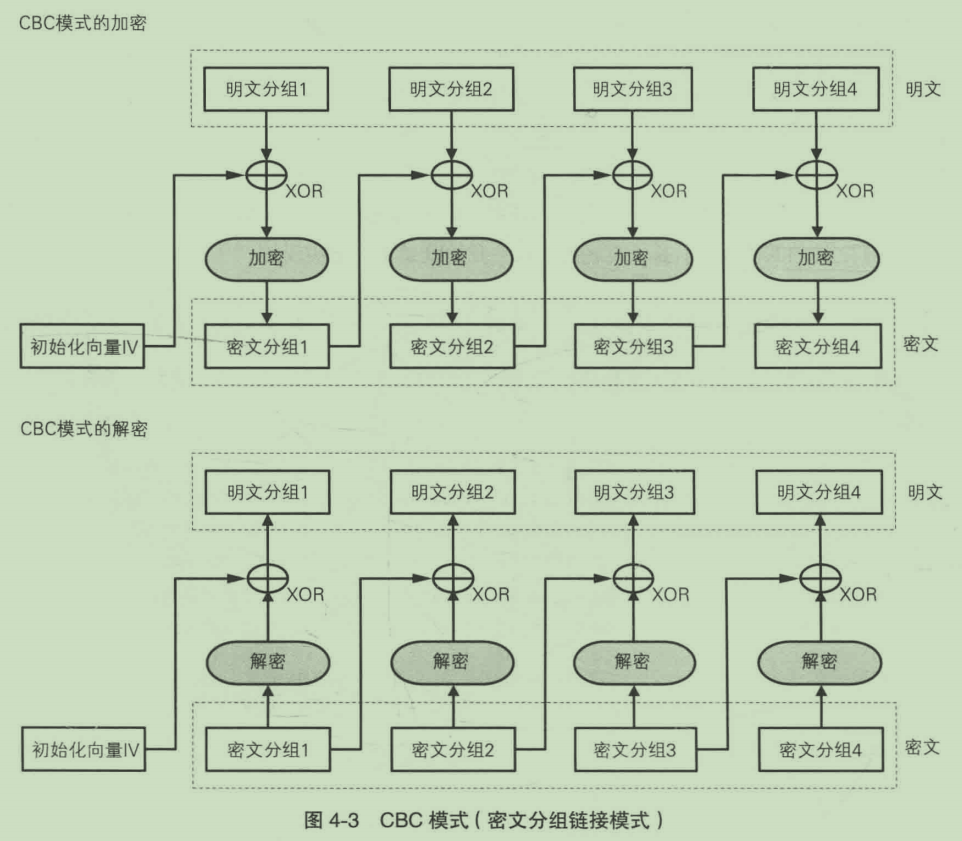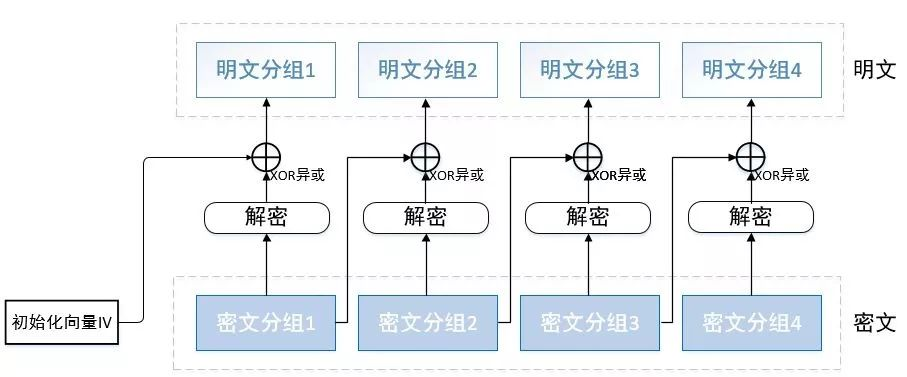# 前言

web题，牵扯到了CBC反转字节攻击，密码学！？

# CBC加解密

CBC 模式中会先将明文分组与前一个密文分组进行 XOR 运算，然后再进行加密。

CBC加解密图示：# 异或的概念

0 ^ 0 = 0
1 ^ 0 = 1
0 ^ 1 = 1
1 ^ 1 = 0

(1) 0 ^ 0 = 0，0 ^ 1 = 1 即 0 异或任何数＝任何数
(2) 1 ^ 0 = 1，1 ^ 1 = 0 即 1 异或任何数 = 任何数取反
(3) 任何数异或自己＝把自己置 0

# CBC反转字节攻击

## 讲解B = A ^ C（解密时）解释：明文分组 3 = 密文分组 2 ^ 解密后的密文分组 3（解密时）
C = A ^ B（加密时）解释： 密文分组 3 = 密文分组 2 ^ 明文分组 3（加密时）

B ^ A ^ C = 0

A ^ B ^ C ^ D = D

## 总结

posted @ 2019-08-13 10:18  yichen0115  阅读(204)  评论(0编辑  收藏